EECS 690: Binary Pulse Amplitude Modulation (PAM)

Objective
The goal of this exercise is to correctly demodulate the noisy received signal in RxBinaryPAM.mat.

Signal Model

The transmitted data points are taken from the signal space constellation below.  Notice the relationship between bits (0, 1) and symbols (-A, +A).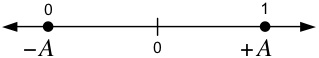After being corrupted by noise, the received signal is demodulated by the system below, which detects the transmitted signal space points and outputs the estimated values.  There are two sample rates in the system below.
The higher sampling rate is 1/T = 16 samples per symbol (indexed by n).  The lower sampling rate is 1/Ts = 1 sample per symbol (indexed by k).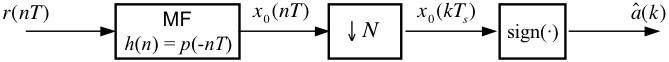Design Exercise

Part I: Binary PAM Modulator

Using blocks from the SIMULINK Block Library, the Signal Processing Blockset, and the Communications Blockset, design a modulator (below) for the system model above.  Use the modulator to send the sequence 1 -1 -1 1.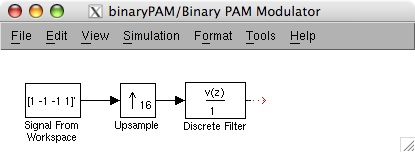The modulator is composed of four blocks.  The first block can be found at
DSP Blockset --> DSP Sources --> Signal From Workspace

Drag this block to your project window.  Double-click on the Signal From Workspace block and set the block parameters as shown in the picture below.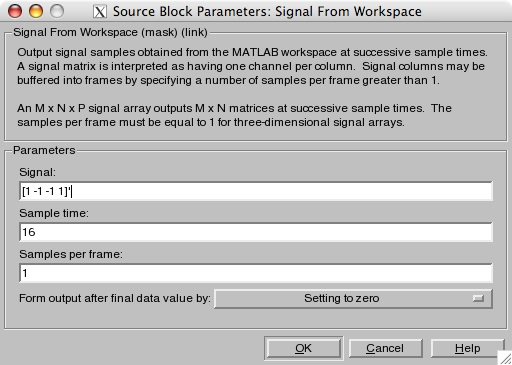The first parameter is a column vector containing the data to be modulated.  The second parameter specifies the sample time (not rate), which is 16 at this point in the system.  We leave the third parameter alone.  The last option (in the drop-down list) indicates what we want to happen when the data runs out but the simulation is still running.  We don't care about this since we will set the simulation time to match the length of the data.

The second block can be found at
DSP Blockset --> Signal Operations --> Upsample

Drag this block to your project window.  Double-click on the Upsample block and set the block parameters as shown in the picture below.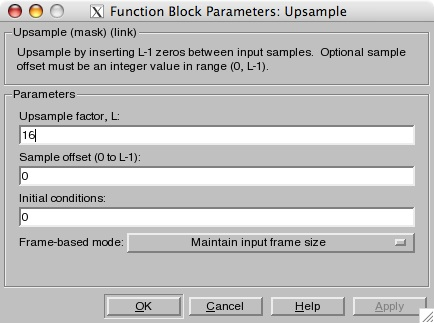d

The first parameter is the upsample factor, which is the number of samples per symbol.  The second parameter is an offset which we do not need to use (at least in the modulator).  Leave it set to the default of zero.

The third block can be found at
Simulink --> Discrete --> Discrete Filter

Drag this block to your project window.  Double-click on the Upsample block and set the block parameters as shown in the picture below.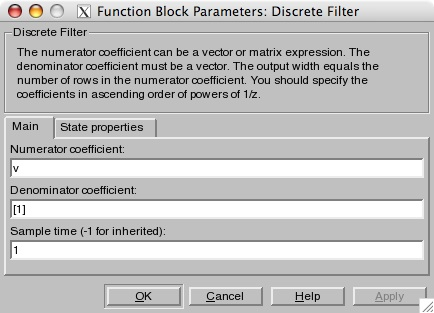e

This block serves as the pulse shaping filter in the modulator.  We want an FIR filter, so we set the numerator to the value of the filter coefficients, and we set the denominator to the trivial vector of.  For the filter coefficents, you can either
1) type sqrt(1/16)*ones(1,16) into the Numerator coefficient box itself, or
2) type v=sqrt(1/16)*ones(1,16) at the Matlab prompt (this makes the images look a little better).
You will recognize this pulse as the NRZ shape sampled at 16 sample per symbol.  The third parameter is the sample time, which is 1 at this point in the system.

Now, test your modulator by connecting the output to the Scope block (this can be found at Simulink --> Sinks).  In your project window, go to the menu Simulation --> Configuration Parameters and set the parameters to:
Start Time:         0.0
Stop Time:          80.0
Solver options:    Type: Fixed-step, Solver: discrete (no continuous states)
Fixed step size:    1
Note: We have to run the simulation for 5x16 samples instead of 4x16 samples since the upsample block inserts a 16 sample delay.  After you go to Simulation --> Run, the output of the scope (double-click the scope) should look like this: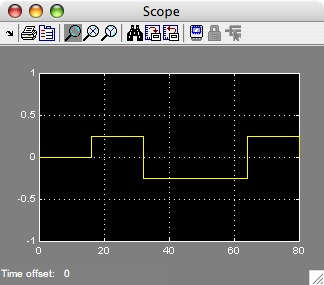Part II: Binary PAM Detector

Design and build a system in SIMULINK that is based on the block diagram of the binary PAM detector shown at the top of this exercise.  The SIMULINK experience you have just gained should prove helpful in this effort.

You can test your detector by connecting its input to the output of your modulator.  Connect the output of your detector to a To Workspace block (this can be found at Simulink --> Sinks, open the Properties and set the Save format to matrix, or array).  After running the simulation, a plot of the matched filter output should look like this: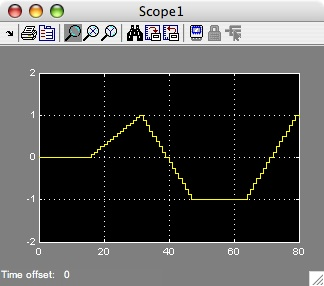You can see with this test input signal (based on the data 1 -1 -1 1), the first valid bit decision occurs at sample 32.  The others occur at samples 48, 64, and 80.  In the Matlab workspace (the Matlab prompt) you will likely find 6 bit decisions instead of 4.  This is because the Downsample block outputs a value at sample 0 and sample 16.  These two extra values can be ignored.

It is important that your Downsample block is sampling at the correct "phase".  This will require some adjustment/experimentation on your part.  You want to sample the matched filter output when the value is exactly +1.0 or -1.0 (think of the eye diagram).  You may need to run the simulation to a stop time of 6*16.0, depending on the sampling offset you use.  When you have successfully debugged your system, you are ready for the final part of the exercise.

Part III: Detecting an Unknown Data Set

Here are the steps for the final part of the exercise:
1. Replace the modulator blocks with the From File block (found at Simulink --> Sources) and set the filename to RxBinaryPAM.mat
2. Set the simulation parameters to:
Start Time:         0.0
Stop Time:          16*(7*10+1)
Solver options:    Type: Fixed-step, Solver: discrete (no continuous states)
Fixed step size:    1
3. Run the Simulation.
4. The last 70 values of the detector output (you will have to convert +/-1s to 1s and 0s) represent 10 ASCII characters, with 7 bits per character.  Determine the message using your own conversion script or an ASCII table, such as the one found here.